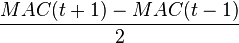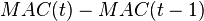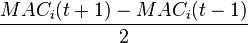# Rate of change of mean age of childbearing

## Definition

The rate of change of mean age of childbearing for a given time interval is an estimate of how the mean age of childbearing has changed from the beginning to the end of that time interval. The time intervals are typically taken to be years. For a year,$t$, if we denote the mean age of childbearing in year$t$ by$MAC(t)$, then we estimate the rate of change in year$t$ by the following formula:$\frac{MAC(t + 1) - MAC(t - 1)}{2}$

Note that this formula can be computed only after an additional year has passed. We could also use the formula:$MAC(t) - MAC(t - 1)$

but this will be less accurate because it is not centered in year$t$.

We can also modify the above formula to consider the mean age of childbearing at a particular birth order. Suppose we denote the mean age of childbearing at birth order$i$ in year$t$ is$MAC_i(t)$. Then, the rate of change is given as:$\frac{MAC_i(t + 1) - MAC_i(t - 1)}{2}$